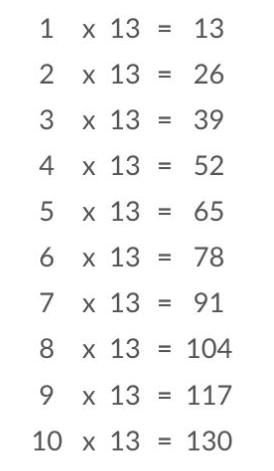# 13 Times Table- Learn Table Of 13 : Multiplication Table Of 13

Safalta expert Published by: Yashaswi More Updated Thu, 18 Aug 2022 11:53 PM IST

## Highlights

Check out how to learn the 13 Times table easily here at Safalta.com

Table of 13 is created by multiplying 13 by whole numbers. Learning is made simpler in high school and beyond by knowing the 13 times tables. Children must master the division facts for the 13-table. For instance, the following are some division facts for the 13-times table: 39 13 = 3, 52 13 = 4, and 65 13 = 5. This will lay the groundwork for dividing greater numbers on a firm basis. Join Safalta School Online and prepare for Board Exams under the guidance of our expert faculty.

### Free Demo ClassesSource: Safalta.com

Our online school aims to help students prepare for Board Exams by ensuring that students have conceptual clarity in all the subjects and are able to score their maximum in the exams.

## 13 Times Table Chart:## Multiplication Table of 13

Table of 13 helps in working out calculations of long multiplication and division having multiples of 13. So, let's see the first 10 multiples of the 13 times table.

## Tips for 13 Times Table

1. To memorize the 13 times table, first, we need to memorize the 3 times table. The multiples of 3 are 3, 6, 9, 12, 15, 18, 21, 24, 27, 30.

2. To obtain the multiples of 13, add natural numbers to the ten's digit of the multiples of 3. Hence, the 13 times table is obtained as follows: (1+0)3, (2+0)6, (3+0)9, (4+1)2, (5+1)5, (6+1)8, (7+2)1, (8+2)4, (9+2)7, (10+3)0 = 13, 26, 39, 52, 65, 78, 91, 104, 117, 130.

3. 13 doesn’t have any rules that make the multiplication table of 13 easy to memorize, but there is a pattern for every ten multiples of three: 13, 26, 39, 52, 65, 78, 91, 104, 117, 130. The last digit of these multiples always repeat, which means that students can remember these digits to help them with the 13 times table.

## Table of 13 up to 20

In the table below, you can see the next 10 multiples of 13, up to 20.

## 13 Times Table Worksheet

1. ### Example 1: If Jack presses the doorbell 1 time, it rings 5 times. Using the table of 13, find how many times does the bell ring when it is pressed 13 times?

Solution:

Number of times the bell rings when pressed once = 5

Therefore, number of times the bell rings when pressed 13 times = 13 times 5 = 65

Hence, the bell will ring 65 times.

2. ### Example 2: If Alex's mother has to pay the amount "13 less than 13 times of 13" in dollars, Using the table if 13 find how much is she required to pay?

Solution:

Mathematically, "13 less than 13 times of 13" = 13 times 13 minus 13

Now, from table of 13, 13 × 13 - 13 = 169 -13 = 156

Hence, Alex's mother needs to pay \$156.

## What is the importance of learning Table 2 to 20?

For making mathematical section easier, memorising table 2 to 20 is important.

## How can I learn tables easily?

Daily recite the table as mentioned in the article twice and thrice.

## What is the 13 times table?

13 Times Table up to 10
13 × 1 = 13 13 × 6 = 78
13 × 2 = 26 13 × 7 = 91
13 × 3 = 39 13 × 8 = 104
13 × 4 = 52 13 × 9 = 117

## What are the factors of 13?

As the number 13 is a prime number, it has only two factors, such as one and the number itself. Thus, the factors of 13 are 1 and 13.